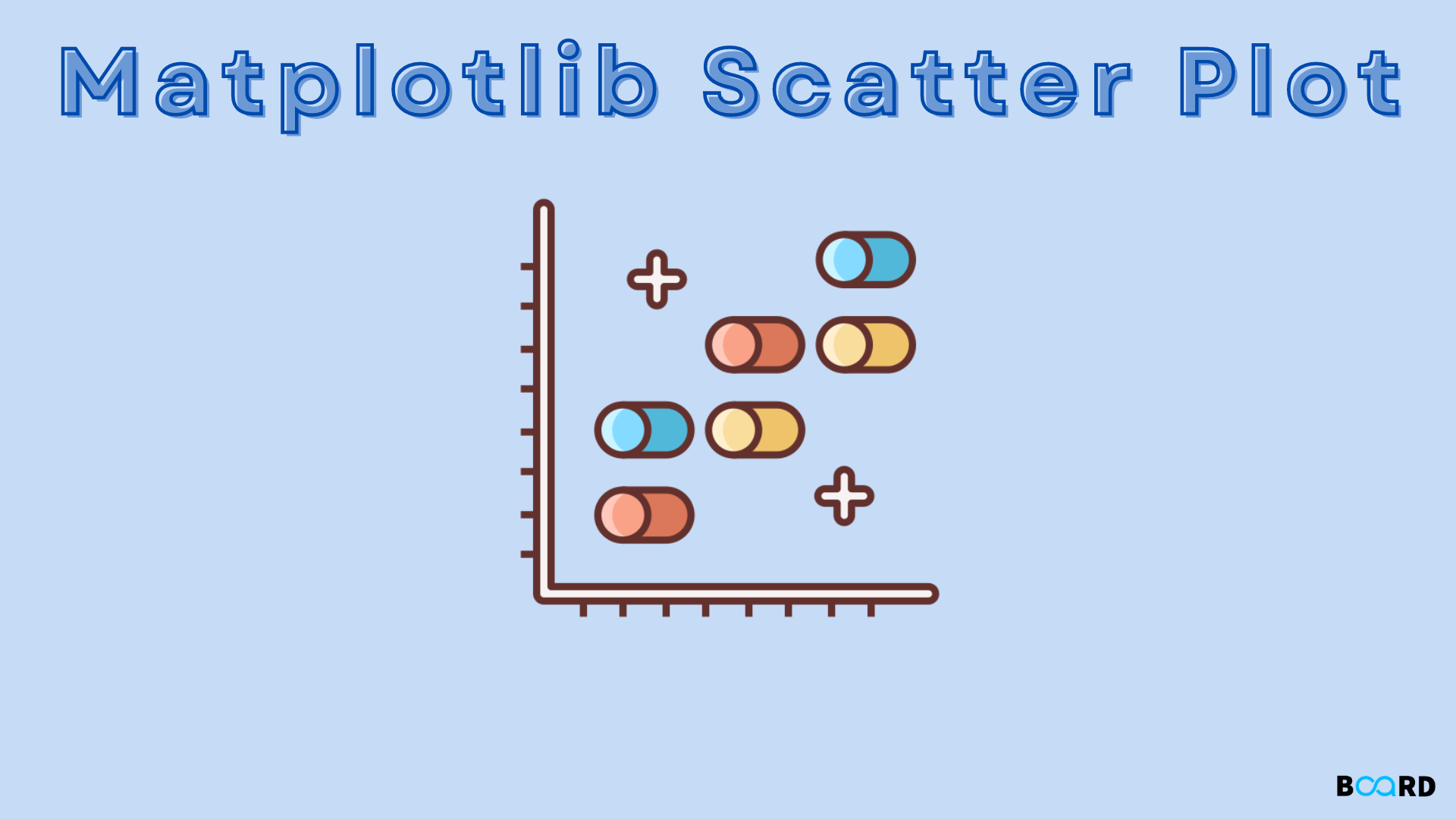# Matplotlib Scatter in Python## Overview

“matplotlib” is a library in Python that enables the creation of interactive, animated, and static visualizations. It may also be used in plotting several plots such as 3-D plots, pie charts, histograms, bar charts, line plots, scatter plots, and many more. We will learn about the scatter plot from the matplotlib library.

Scatter plots are required for observing the relationship among variables using dots. The “scatter” function of the library matplotlib can be used for drawing scatter plots which are used in representing relationships among variables and how a change in one relationship affects another.

## Scope

• Syntax of using matplotlib.pyplot.scatter() in Python
• Examples with code implementation.

## Syntax

The code block ahead depicts the usage of the method matplotlib.pyplot.scatter():

``````matplotlib.pyplot.scatter(xAxisData, yAxisData, s=None, c=None, marker=None, cmap=None, vmin=None, vmax=None, alpha=None, linewidths=None, edgecolors=None)
``````

## Parameters

1. xAxisData: It’s an array that contains the x-axis data.
2. yAxisData: It’s an array that contains the y-axis data.
3. s: denotes the value of the marker size.
4. c: It’s the sequence of colors for markers.
5. marker: The style of the marker.
6. cmap: The name of the cmap.
7. linewidths: Denotes the width of the border of the marker.
8. edgecolor: Represents the color of the border of the marker.
9. alpha: The blending value.

All arguments, Except xAxisData and yAxisData are obligatory, and their default value is None.

## Examples

### Example 1

``````import matplotlib.pyplot as mplpp
x = [15, 70, 18, 70, 22, 17, 12, 9, 24, 14, 14, 49, 27]
y = [51, 68, 43, 22, 0, 23, 123, 67, 32, 89, 75, 90, 88]
mplpp.scatter(x, y, c ="orange")
mplpp.show()``````

Output

### Example 2

``````import matplotlib.pyplot as mplpp
x1 = [112, 34, 23, 21, 78, 0, 2, 23, 89, 34]
y1 = [123, 132, 45, 54, 78, 3, 261, 165, 76, 92]
x2 = [31, 23, 67, 52, 5, 32, 83, 29, 38, 64]
y2 = [26, 34, 90, 33, 38, 20, 56, 2, 47, 15]
mplpp.scatter(x1, y1, c ="red", linewidths = 2, marker ="s", edgecolor ="blue>
mplpp.scatter(x2, y2, c ="grey", linewidths = 2, marker ="^", edgecolor ="pur>
mplpp.xlabel("x-axis")
mplpp.ylabel("y-axis")
mplpp.show()
``````

Output